## Bayesian longitudinal/panel-data models

You can fit Bayesian longitudinal/panel-data models by simply prefixing your classical panel-data models with bayes:. Because panel-data models can be viewed as two-level hierarchical models, all the benefits of Bayesian multilevel modeling apply to panel-data models too.

You can fit a linear random-effects panel-data model to outcome y with predictors x1 and x2 and panel or group identifier id by typing

`. xtset id . xtreg y x1 x2 `

You can now fit a Bayesian counterpart of this model by typing

```. xtset id
. bayes: xtreg y x1 x2
```

Of course, as with any other Stata command, you can use a point-and-click interface instead of typing the commands.

Bayesian panel-data models are not only for continuous outcomes. You can just as easily type for binary outcomes

`. bayes: xtprobit y x1 x2 `

or for count outcomes

`. bayes: xtpoisson y x1 x2 `

Or use any of the eight panel-data models that support the bayes prefix, including the new panel-data multinomial logit model.

## Let’s see it work

Estimation

Convergence of MCMC

Custom priors

Posterior distributions of panel or group effects

Bayesian predictions

Posterior predictive checks—model fit

Clean up

## Estimation

Consider a subset of data from the National Longitudinal Survey of Young Women between 14 and 24 years old in 1968, living in the South. We will model the log of wages as a function of individual’s education, grade; their work experience, ttl_exp, which enters the model quadratically; and whether or not they live in a standard metropolian area, not_smsa. There are multiple observations per individual, identified by id.

We fit a Bayesian panel-data linear model to account for individual effects. We also wish to compute Bayesian predictions of log wages and compare individuals’ (panel) effects.

```. webuse nlswork6
(Subsample of 1986 National Longitudinal Survey of Young Women)
```

To save time, we run a Markov chain Monte Carlo (MCMC) sample of 1,000, instead of the default 10,000. We also specify a random-number seed for reproducibility.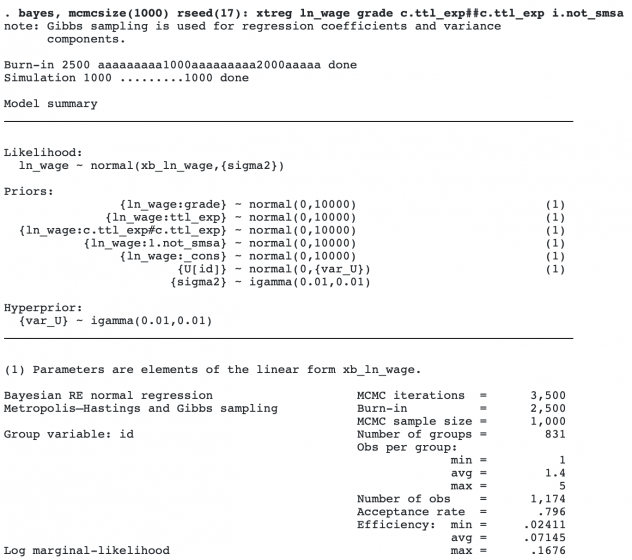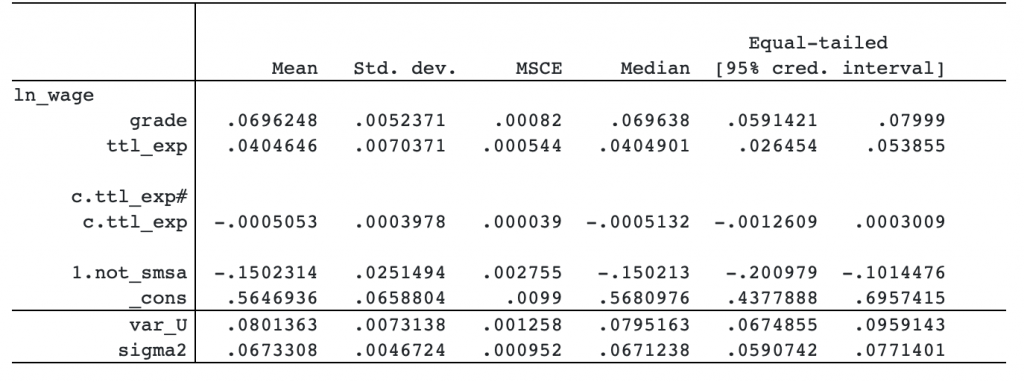The posterior mean for the grade coefficient is positive, with a magnitude of 7 percent. Theory suggests that wages increase with experience but the increase tapers with time. This implies that the coefficient for ttl_exp should be positive, and the coefficient for c.ttl_exp#c.ttl_exp should be negative. We observe this in our data. Finally, living outside big urban centers has a negative effect on wages.

Bayesian modeling requires that you specify priors for all model parameters. As with any other bayes command, bayes: xtreg provides default priors for convenience. You should review the prior specifications and specify your own.

## Convergence of MCMC

bayes: xtreg uses MCMC to obtain results. Its convergence needs to be verified before further analysis. You can do this graphically, or you can compute the Gelman–Rubin convergence statistic using multiple chains.

Let’s assess MCMC convergence visually for, say, the grade coefficient.

```. bayesgraph diagnostics {ln_wage:grade}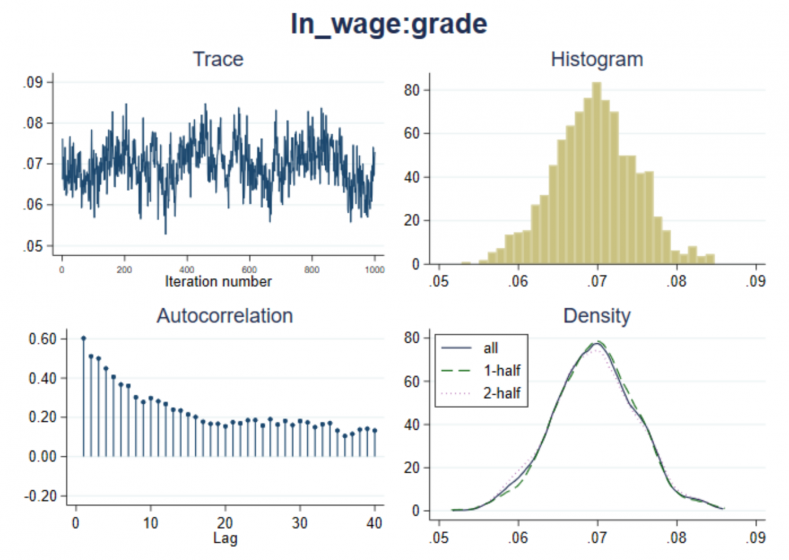```

There is no apparent trend in the trace plot. Autocorrelation decreases with time. We do not have a reason to suspect nonconvergence for grade, but convergence needs to be verified for all model parameters.

Let’s save our MCMC and estimation results for later use.

```. bayes, saving(bxtregsim)
note: file bxtregsim.dta saved.

. estimates store bxtreg
```

## Custom priors

With Bayesian models, you may want to incorporate your own priors. These priors often come from historical data. For instance, based on previous studies, it may be reasonable to assume that the coefficient for grade ranges between 0 and 25. So we may consider a uniform on (0,25) prior for it. We can use bayes‘s option prior() to specify custom priors.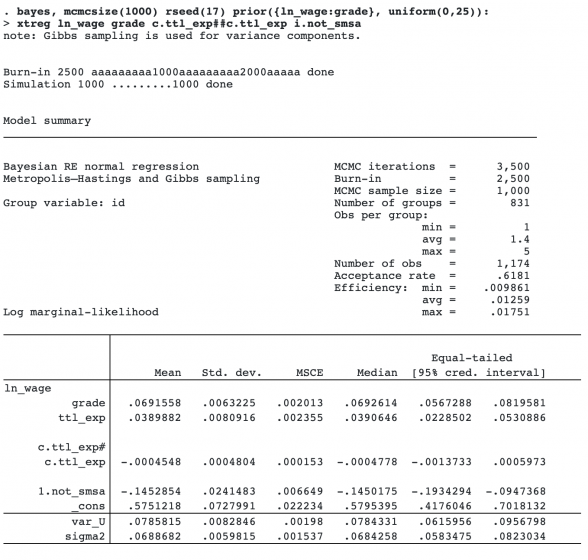Our custom prior did not change the results much.

## Posterior distributions of panel or group effects

We may be interested in making inference about individuals’ (panel) effects. Bayesian modeling provides a natural way of doing this. Unlike classical random-effects panel-data models, Bayesian panel-data models estimate random (panel) effects together with all other model parameters. As such, each random effect is represented by an entire MCMC sample from its posterior distribution. This sample can be used for inference such as comparison of panel or group effects. (Think of comparing performances of different companies or hospitals.)

Let’s return to our original model, which used default uninformative priors.

```. estimates restore bxtreg
(results bxtreg are active now)
```

Let’s plot the posterior distributions for the first nine individual effects.

```. bayesgraph histogram {U[1/9]}, byparm normal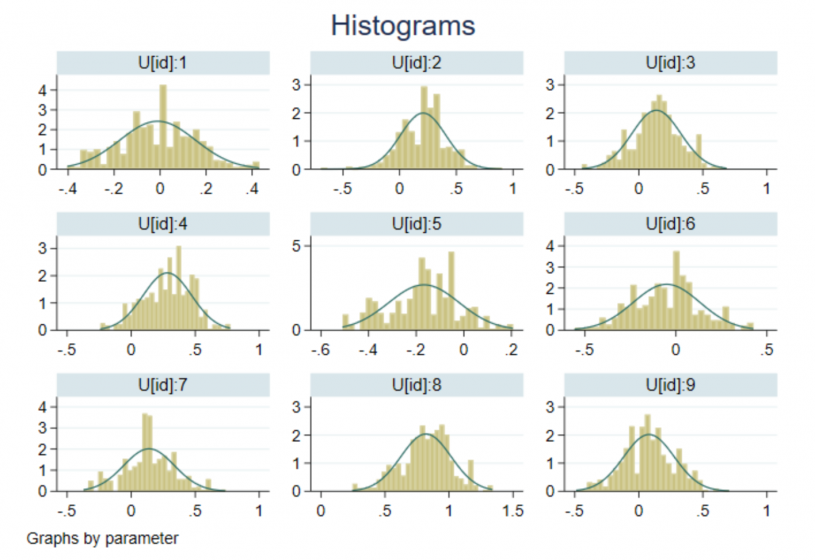```

The above individual effects represent shifts or offsets from the average log wage. There is definitely variation among individuals’ salaries. For instance, we can see that the salary of individual 8 is higher than that of individual 5. So there are still differences between individual salaries that are not accounted for by the model predictors.

## Bayesian predictions

Within the Bayesian framework, you can compute predictions and their uncertainties without making any asymptotic assumptions. This is possible because the obtained predictions are samples from the “exact” posterior predictive distribution of the new data given the observed data. The posterior predictive distribution is not assumed to be asymptotically normal.

Let’s compute posterior means of predicted log wages together with their 95% credible intervals.

```. bayespredict pmean, mean

Computing predictions ...

. bayespredict cri_l cri_u, cri

Computing predictions ...
```

Let’s list the results for the first 10 observations.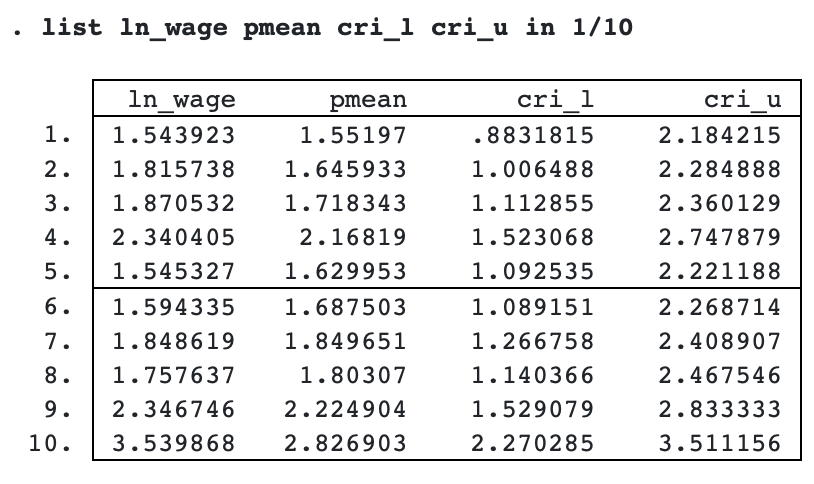The predicted posterior means are close to the observed values except perhaps for observation 10. In the next section, we show how to check whether the model fits well more formally. But to do so, we must first save all MCMC predictions.

```. bayespredict {_ysim1}, saving(bxtregpred)

Computing predictions ...

file bxtregpred.dta saved.
file bxtregpred.ster saved.
```

bxtregpred.dta contains an MCMC sample of values for each observation. The posterior means (pmean) we predicted earlier are, for each observation, the mean over the MCMC sample.

The MCMC predictions datasets are typically large, so you may consider saving them only when necessary; see [BAYES] bayespredict for details.

## Posterior predictive checks—model fit

Another advantage of Bayesian analysis of panel-data models is formalized posterior predictive checks for checking model fit that are not available with classical frequentist analysis.

We can use posterior samples for the predicted outcome, the MCMC prediction sample, to perform model goodness-of-fit checks. For example, let’s compare the minimum and maximum statistics from the MCMC prediction samples with those observed in the data. These statistics describe the tails of the data distribution. You can use any other statistics in place of (or in addition to) the maximum and minimum.

bayesstats ppvalues performs posterior predictive checks by computing the so-called posterior predictive p-values (PPPs). PPPs describe how often the statistics from the MCMC prediction sample were as extreme as or more extreme than those in the observed sample; see [BAYES] bayesstats ppvalues.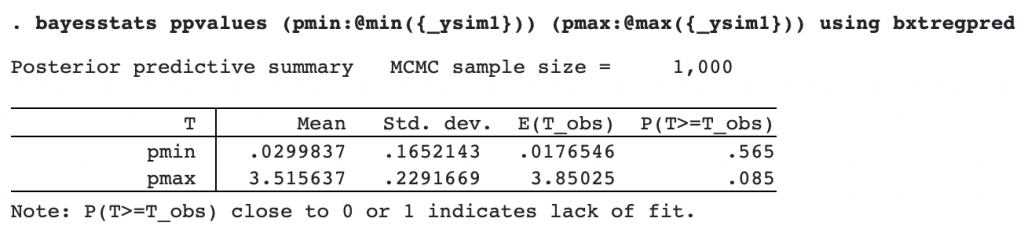The PPP for the minimum, .565, is not close to 0 or 1, so the model fits well with respect to this statistic. But the PPP for the maximum, .085, is close to 0 and thus suggests a poor fit for this statistic.

If we examine the data, we will discover a cluster of observations with values between 3.5 and 4 that look like outliers. We may need to revisit our model and address the issue of outliers.

## Clean up

After the analysis, we can remove the files created by bayes: var and bayespredict that are no longer needed.

```. erase bxtregsim.dta
. erase bxtregpred.dta
. erase bxtregpred.ster
```